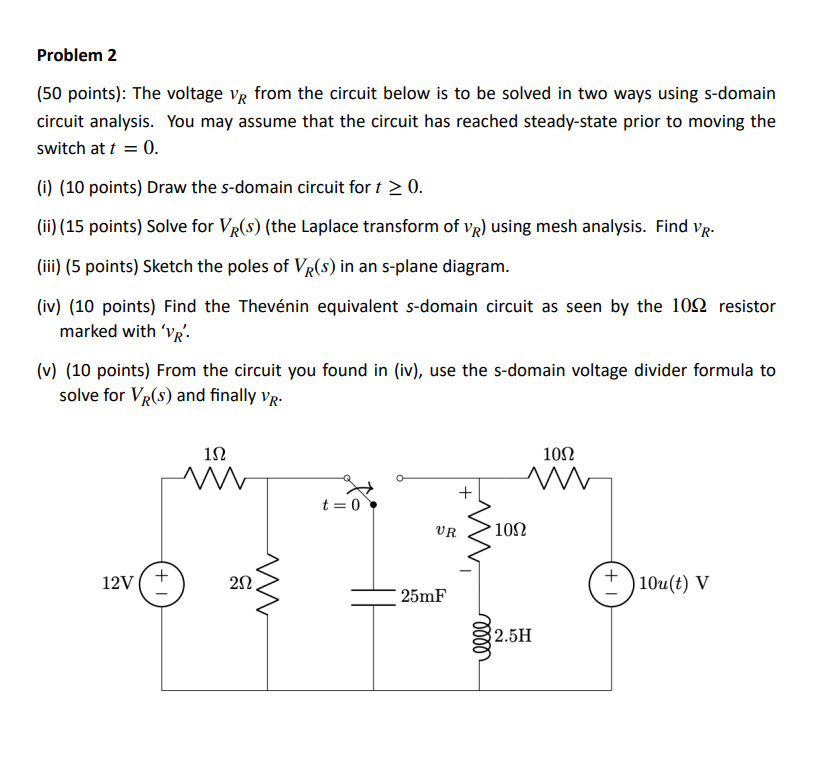Home / Expert Answers / Electrical Engineering / 50-points-the-voltage-vr-from-the-circuit-below-is-to-be-solved-in-two-ways-using-s-domain-ci-pa346

# (Solved): (50 points): The voltage vR from the circuit below is to be solved in two ways using s-domain ci ...(50 points): The voltage from the circuit below is to be solved in two ways using s-domain circuit analysis. You may assume that the circuit has reached steady-state prior to moving the switch at . (i) (10 points) Draw the -domain circuit for . (ii) (15 points) Solve for (the Laplace transform of ) using mesh analysis. Find . (iii) (5 points) Sketch the poles of in an s-plane diagram. (iv) (10 points) Find the Thevénin equivalent -domain circuit as seen by the resistor marked with ' '. (v) (10 points) From the circuit you found in (iv), use the s-domain voltage divider formula to solve for and finally .

We have an Answer from Expert